### Discover more content...

Enter some keywords in the search box above, we will do our best to offer you relevant results.

### We're sorry!

We couldn't find any results for your search. Please try again with another keywords.

##### Tags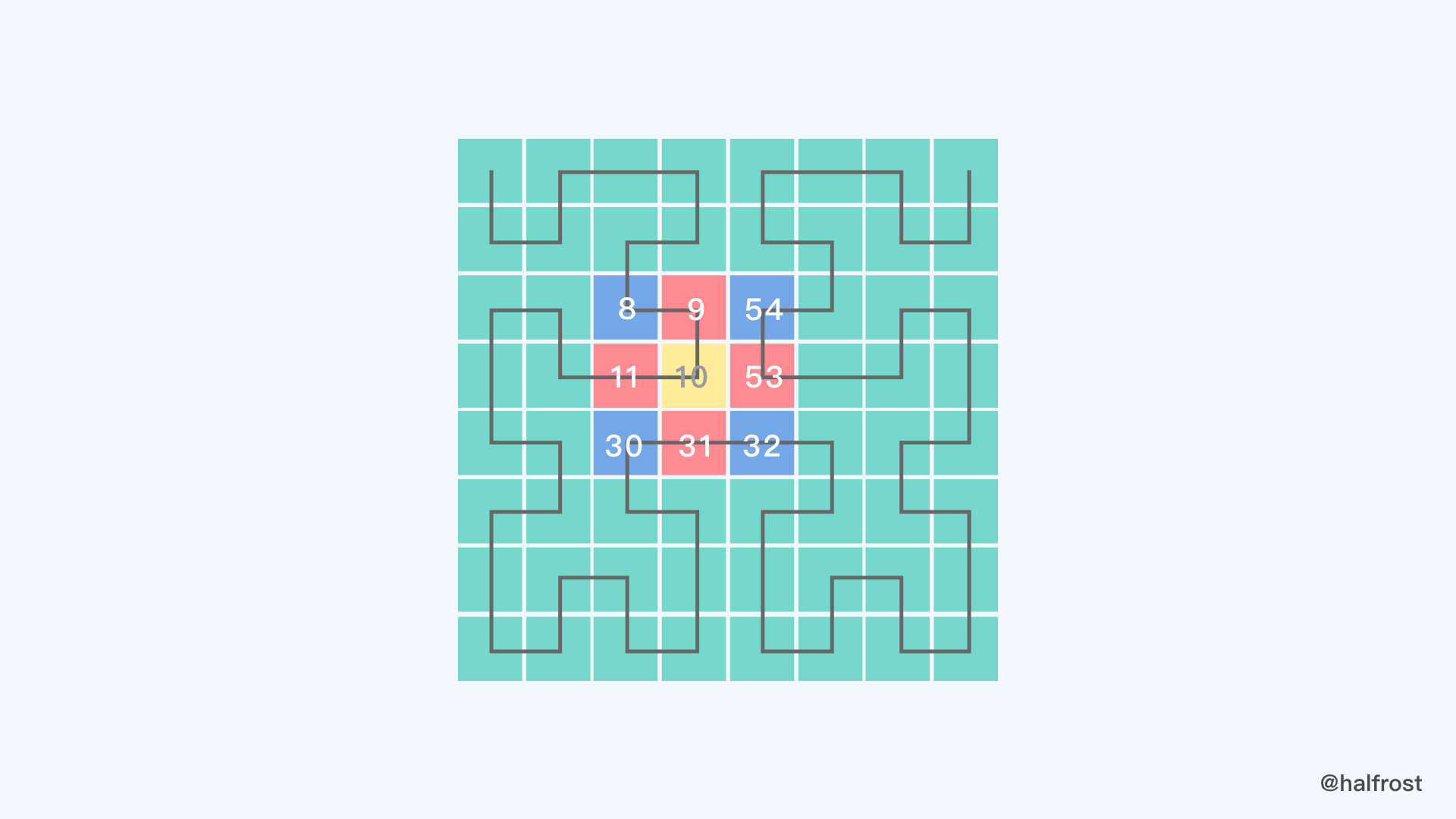## 一. 边邻居

``````

func (ci CellID) EdgeNeighbors() CellID {
level := ci.Level()
size := sizeIJ(level)
f, i, j, _ := ci.faceIJOrientation()
return CellID{
cellIDFromFaceIJWrap(f, i, j-size).Parent(level),
cellIDFromFaceIJWrap(f, i+size, j).Parent(level),
cellIDFromFaceIJWrap(f, i, j+size).Parent(level),
cellIDFromFaceIJWrap(f, i-size, j).Parent(level),
}
}

``````

``````
func sizeIJ(level int) int {
return 1 << uint(maxLevel-level)
}

``````

sizeIJ 保存的是当前 Level 的格子边长大小。这个大小是相对于 Level 30 来说的。比如 level = 29，那么它的 sizeIJ 就是2，代表 Level 29 的一个格子边长是由2个 Level 30 的格子组成的，那么也就是2^2^=4个小格子组成的。如果是 level = 28，那么边长就是4，由16个小格子组成。其他都以此类推。

``````
func (ci CellID) faceIJOrientation() (f, i, j, orientation int) {

f = ci.Face()
nbits := maxLevel - 7*lookupBits // first iteration

for k := 7; k >= 0; k-- {
orientation += (int(uint64(ci)>>uint64(k*2*lookupBits+1)) & ((1 << uint((2 * nbits))) - 1)) << 2
orientation = lookupIJ[orientation]
i += (orientation >> (lookupBits + 2)) << uint(k*lookupBits)
j += ((orientation >> 2) & ((1 << lookupBits) - 1)) << uint(k*lookupBits)
nbits = lookupBits // following iterations
}
// 下面这个判断详细解释
if ci.lsb()&0x1111111111111110 != 0 {
}
return
}

``````

cellIDFromFaceIJ 是把 face，i，j 这个当入参传进去，返回值是 CellID，faceIJOrientation 是把 CellID 分解成 face，i，j，orientation。faceIJOrientation 比 cellIDFromFaceIJ 分解出来多一个 orientation。

``````
func cellIDFromFaceIJWrap(f, i, j int) CellID {
// 1.
i = clamp(i, -1, maxSize)
j = clamp(j, -1, maxSize)

// 2.
const scale = 1.0 / maxSize
limit := math.Nextafter(1, 2)
u := math.Max(-limit, math.Min(limit, scale*float64((i<<1)+1-maxSize)))
v := math.Max(-limit, math.Min(limit, scale*float64((j<<1)+1-maxSize)))
// 3.
f, u, v = xyzToFaceUV(faceUVToXYZ(f, u, v))
return cellIDFromFaceIJ(f, stToIJ(0.5*(u+1)), stToIJ(0.5*(v+1)))
}

``````

``````
func clamp(x, min, max int) int {
if x < min {
return min
}
if x > max {
return max
}
return x
}

``````

clamp 函数就是用来限定 i ， j 的范围的。i，j 的范围始终限定在 [-1，maxSize] 之间。

``````
func stToUV(s float64) float64 {
if s >= 0.5 {
return (1 / 3.) * (4*s*s - 1)
}
return (1 / 3.) * (1 - 4*(1-s)*(1-s))
}

``````

``````
u = 2 * s - 1
v = 2 * t - 1

``````

u，v 的取值范围都被限定在 [-1，1] 之间。具体代码实现：

``````
const scale = 1.0 / maxSize
limit := math.Nextafter(1, 2)
u := math.Max(-limit, math.Min(limit, scale*float64((i<<1)+1-maxSize)))
v := math.Max(-limit, math.Min(limit, scale*float64((j<<1)+1-maxSize)))

``````

``````
f, u, v = xyzToFaceUV(faceUVToXYZ(f, u, v))
return cellIDFromFaceIJ(f, stToIJ(0.5*(u+1)), stToIJ(0.5*(v+1)))

``````

``````
return CellID{
cellIDFromFaceIJWrap(f, i, j-size).Parent(level),
cellIDFromFaceIJWrap(f, i+size, j).Parent(level),
cellIDFromFaceIJWrap(f, i, j+size).Parent(level),
cellIDFromFaceIJWrap(f, i-size, j).Parent(level),
}

``````

``````

3958603599319138304 // 下边邻居
3958607997365649408 // 右边邻居
3958612395412160512 // 上边邻居
3958599201272627200 // 左边邻居

``````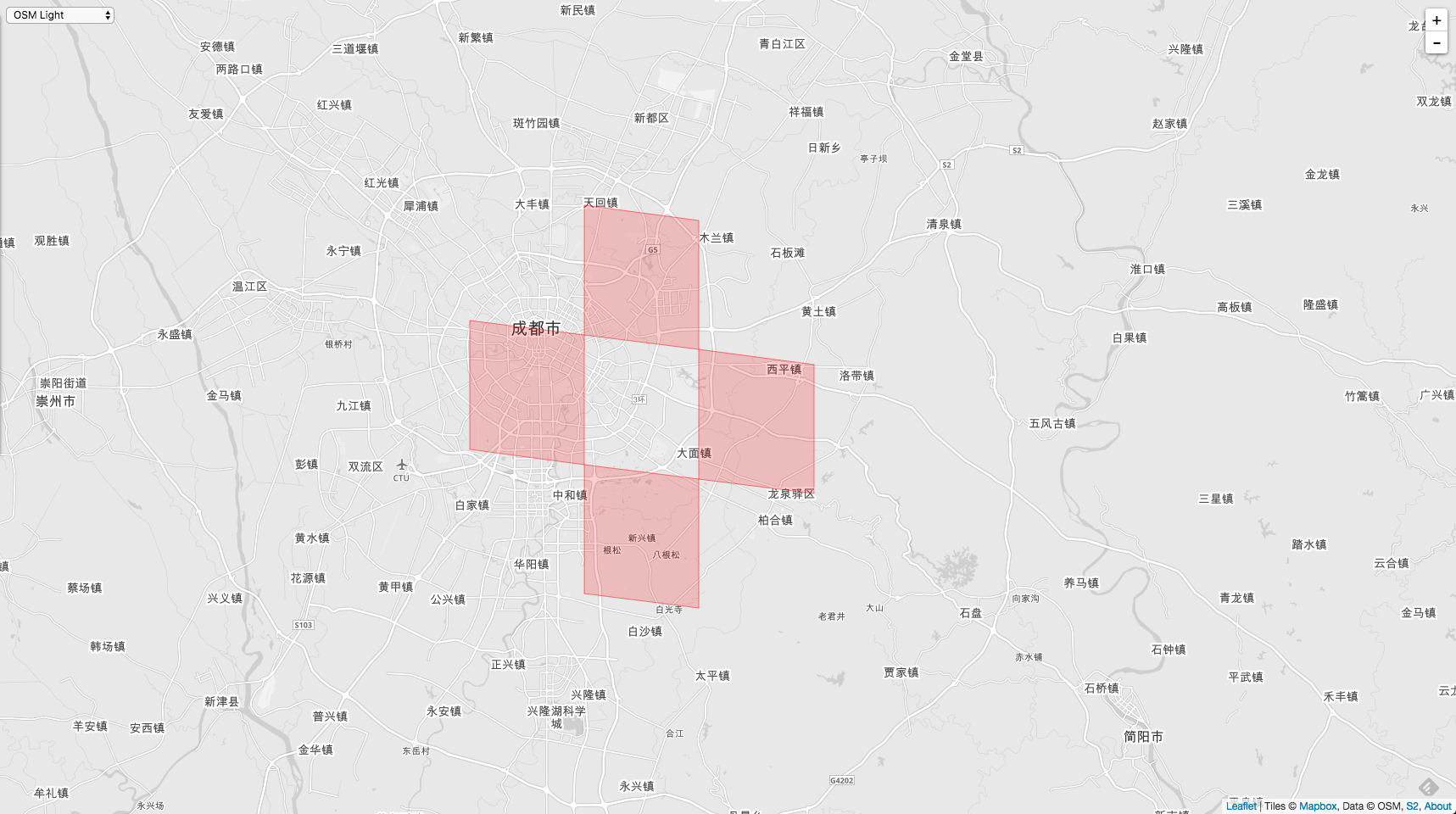## 二. 共顶点邻居

``````
j
|
|  (0,1)  (1,1)
|  (0,0)  (1,0)
|
---------------> i

``````

``````
halfSize := sizeIJ(level + 1)
size := halfSize << 1
f, i, j, _ := ci.faceIJOrientation()

var isame, jsame bool
var ioffset, joffset int

``````

``````
if i&halfSize != 0 {
// 位于后边一列，所以偏移量要加上一个格子
ioffset = size
isame = (i + size) < maxSize
} else {
// 位于左边一列，所以偏移量要减去一个格子
ioffset = -size
isame = (i - size) >= 0
}

``````

j 轴判断原理和 i 完全一致。

``````
if j&halfSize != 0 {
// 位于上边一行，所以偏移量要加上一个格子
joffset = size
jsame = (j + size) < maxSize
} else {
// 位于下边一行，所以偏移量要减去一个格子
joffset = -size
jsame = (j - size) >= 0
}

``````

``````

results := []CellID{
ci.Parent(level),
cellIDFromFaceIJSame(f, i+ioffset, j, isame).Parent(level),
cellIDFromFaceIJSame(f, i, j+joffset, jsame).Parent(level),
}

``````

``````
if isame || jsame {
results = append(results, cellIDFromFaceIJSame(f, i+ioffset, j+joffset, isame && jsame).Parent(level))
}

``````

``````
func (ci CellID) VertexNeighbors(level int) []CellID {
halfSize := sizeIJ(level + 1)
size := halfSize << 1
f, i, j, _ := ci.faceIJOrientation()

fmt.Printf("halfsize 原始的值 = %v-%b\n", halfSize, halfSize)
var isame, jsame bool
var ioffset, joffset int

if i&halfSize != 0 {
// 位于后边一列，所以偏移量要加上一个格子
ioffset = size
isame = (i + size) < maxSize
} else {
// 位于左边一列，所以偏移量要减去一个格子
ioffset = -size
isame = (i - size) >= 0
}
if j&halfSize != 0 {
// 位于上边一行，所以偏移量要加上一个格子
joffset = size
jsame = (j + size) < maxSize
} else {
// 位于下边一行，所以偏移量要减去一个格子
joffset = -size
jsame = (j - size) >= 0
}

results := []CellID{
ci.Parent(level),
cellIDFromFaceIJSame(f, i+ioffset, j, isame).Parent(level),
cellIDFromFaceIJSame(f, i, j+joffset, jsame).Parent(level),
}

if isame || jsame {
results = append(results, cellIDFromFaceIJSame(f, i+ioffset, j+joffset, isame && jsame).Parent(level))
}

return results
}

``````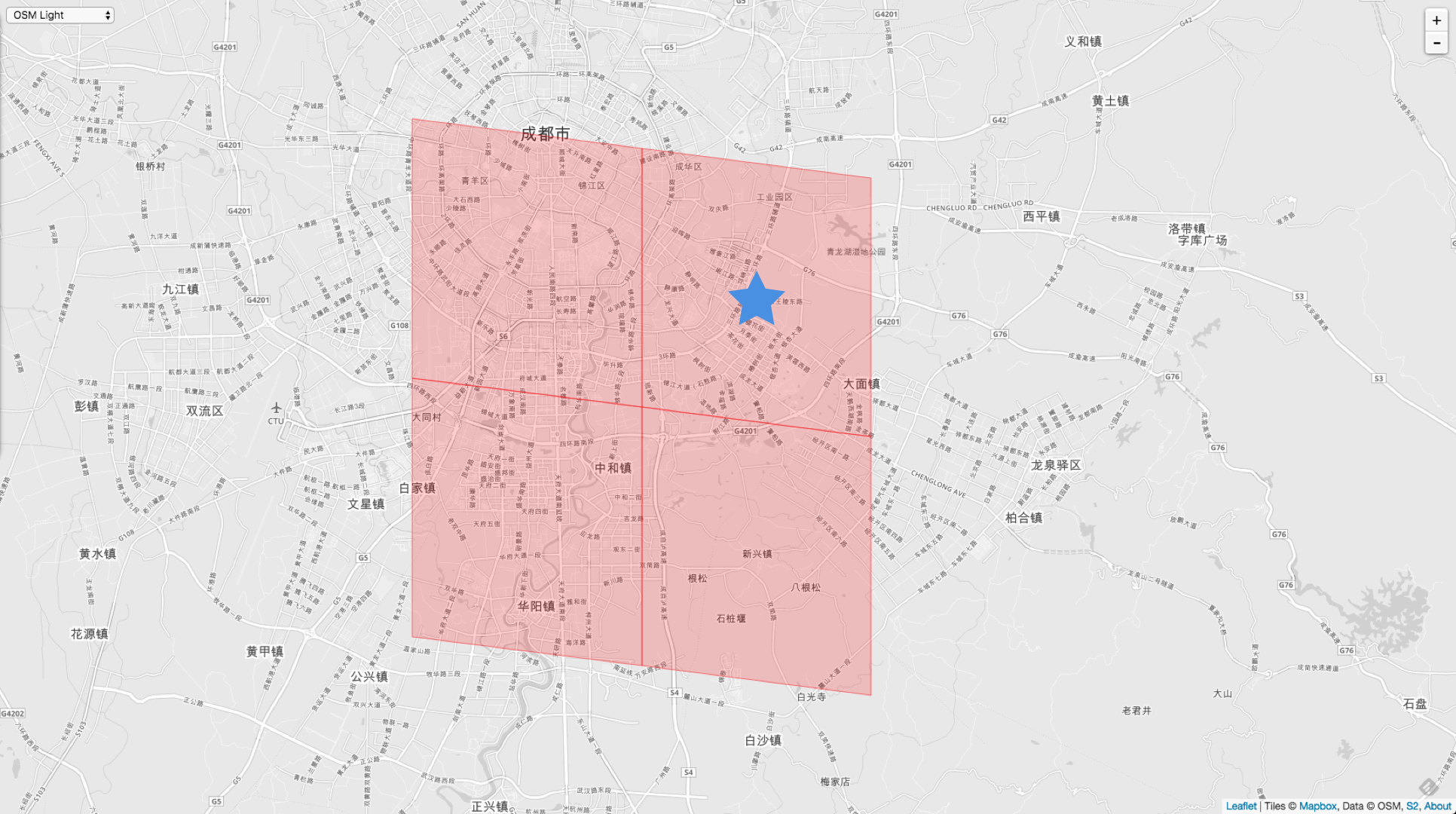``````
VertexNeighbors := cellID.Parent(10).VertexNeighbors(10)

// 11011011101111110011110000000000000000000000000000000000000000
3958610196388904960 // 右上角
3958599201272627200 // 左上角
3958603599319138304 // 右下角
3958601400295882752 // 左下角

``````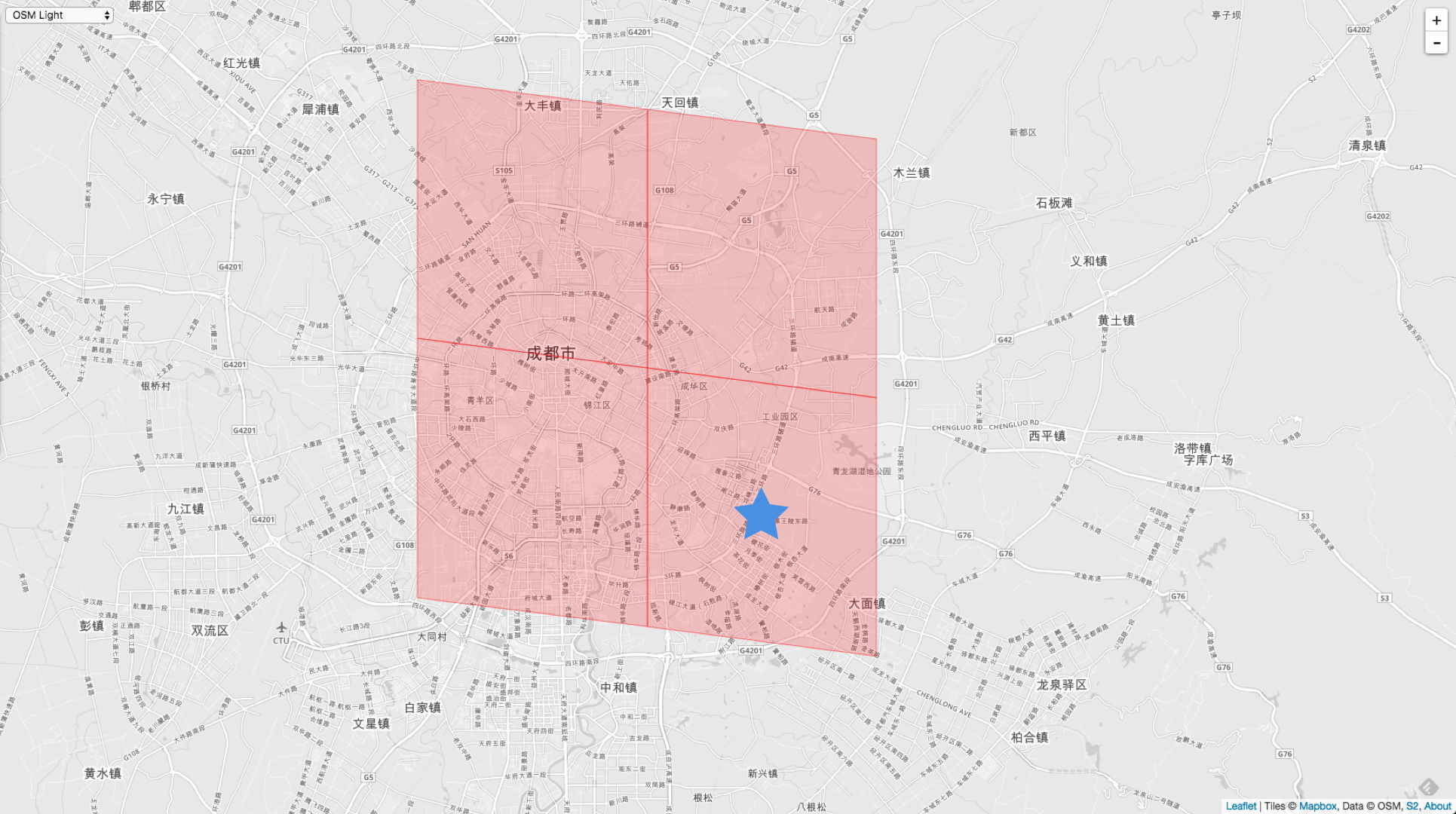``````
VertexNeighbors := cellID.VertexNeighbors(10)

// 11011011101111110011110000000000000000000000000000000000000000
3958610196388904960 // 右下角
3958599201272627200 // 左下角
3958612395412160512 // 右上角
3958623390528438272 // 左上角

``````

``````
DCHECK_LT(level, this->level());

``````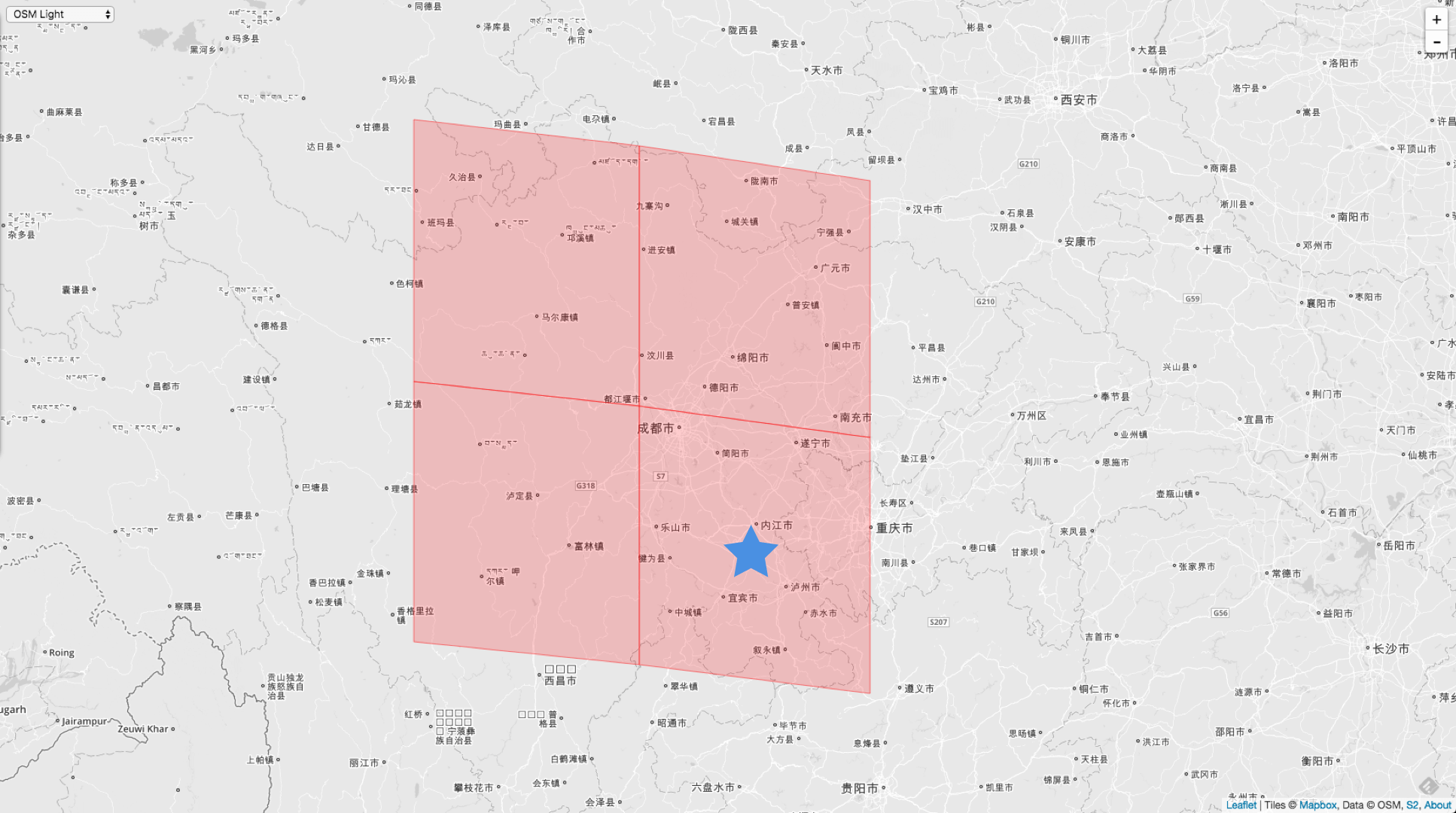``````
VertexNeighbors := cellID.Parent(10).VertexNeighbors(5)

3957538172551823360 // 右下角
3955286372738138112 // 左下角
3959789972365508608 // 右上角
3962041772179193856 // 左上角

``````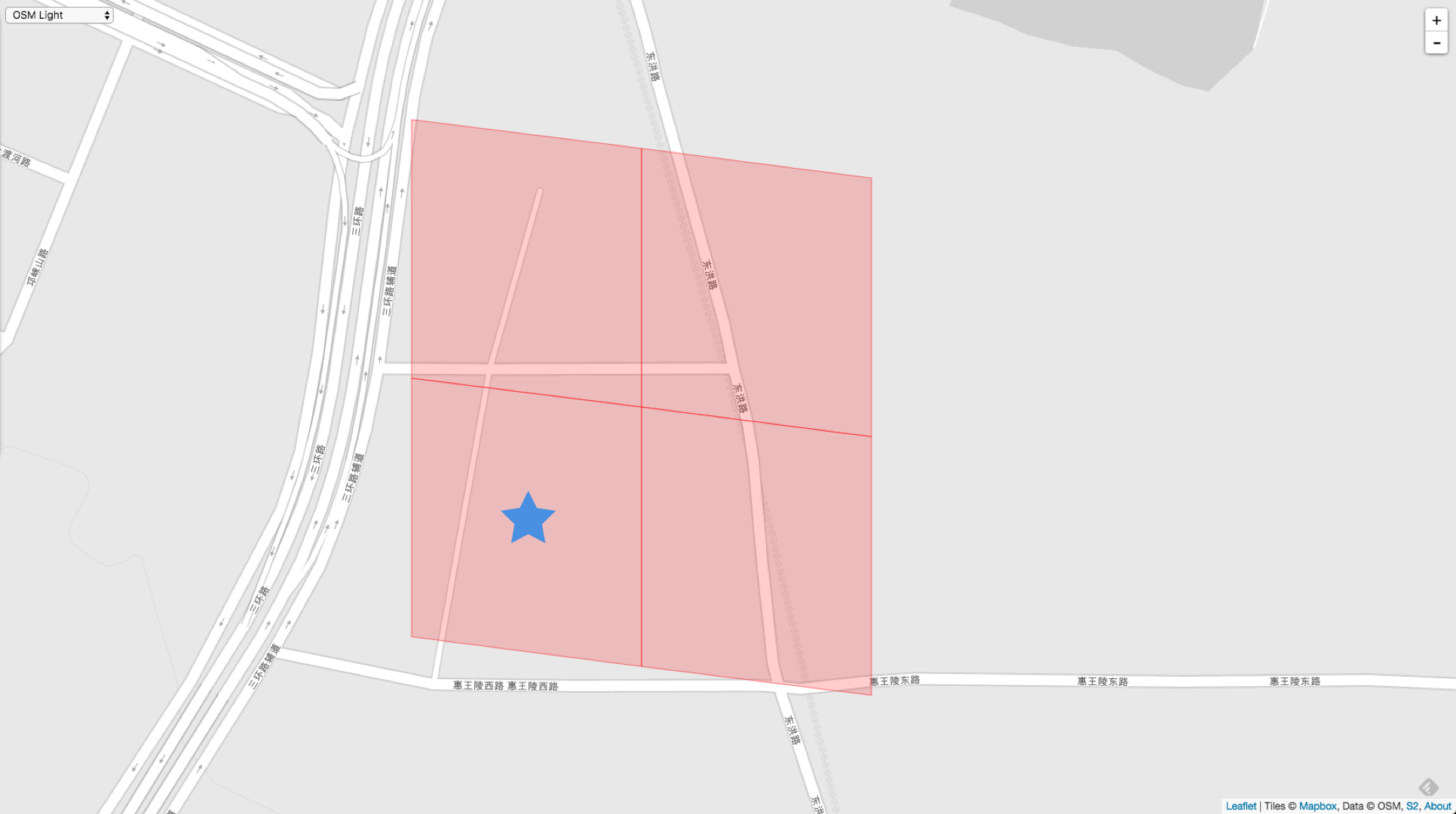``````
VertexNeighbors := cellID.Parent(10).VertexNeighbors(15)

3958610197462646784 // 左下角
3958610195315163136 // 右下角
3958610929754570752 // 左上角
3958609463023239168 // 右上角

``````

## 三. 全邻居

``````
AllNeighbors := cellID.Parent(10).AllNeighbors(5)

``````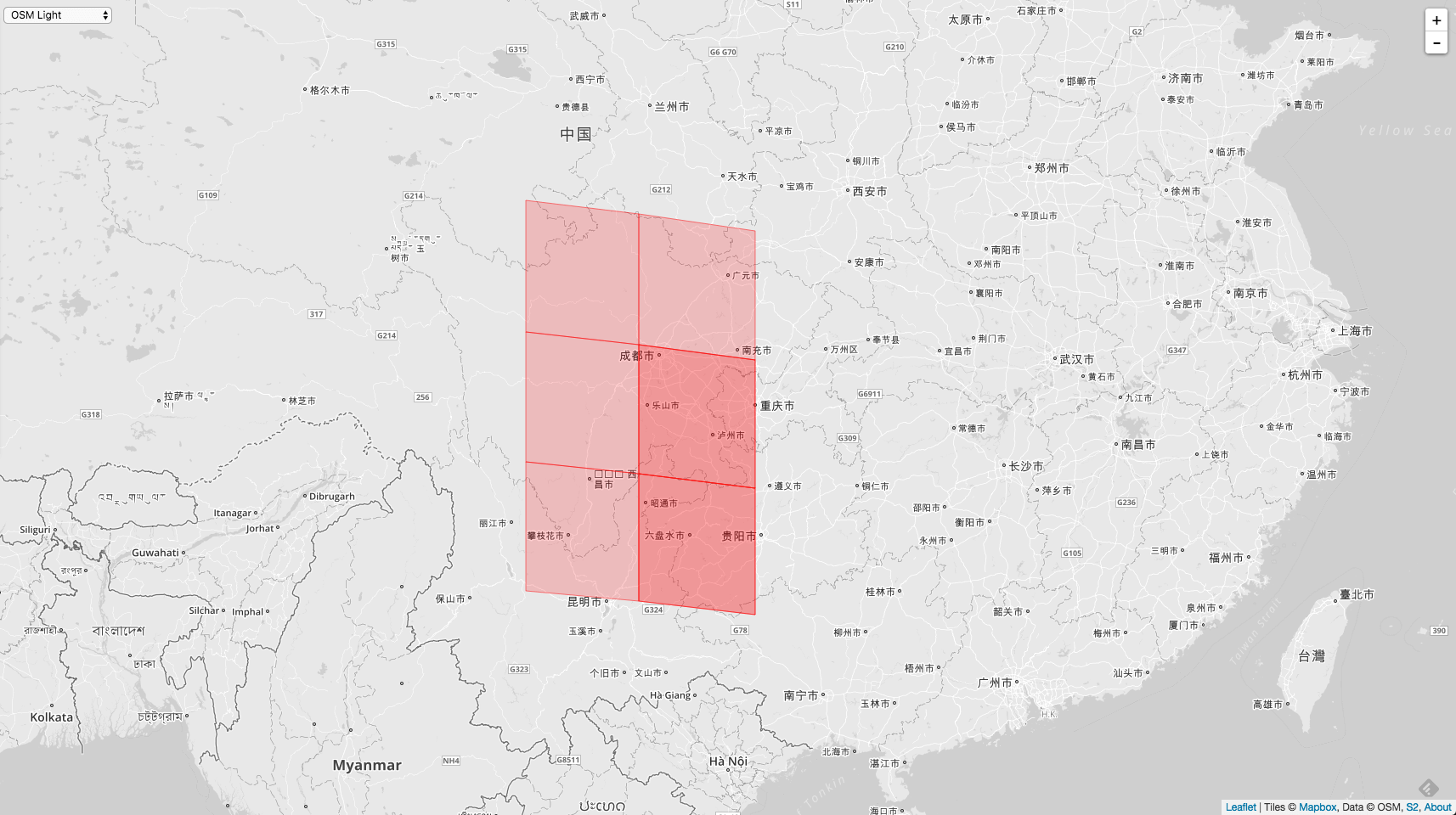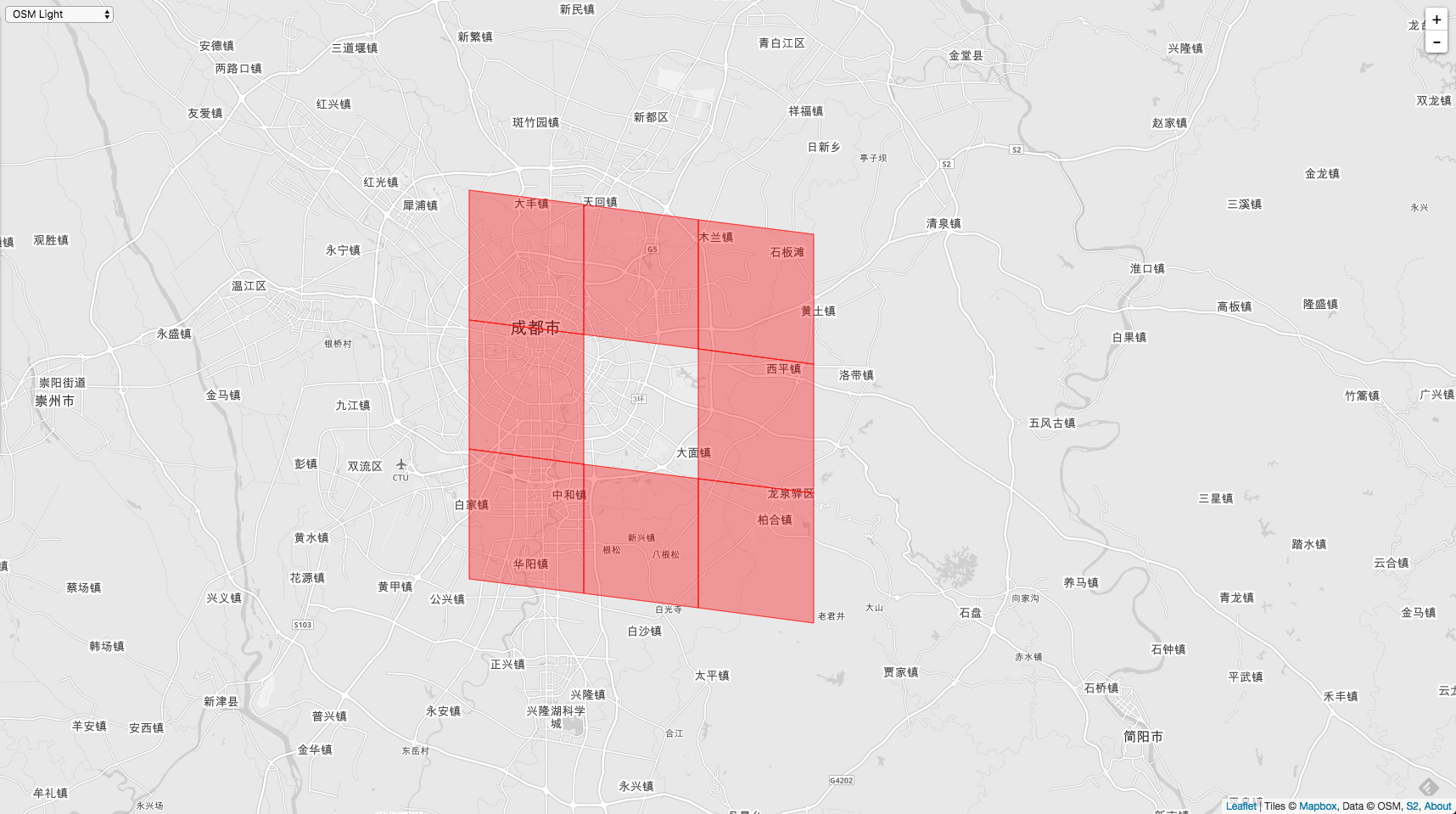``````
func (ci CellID) AllNeighbors(level int) []CellID {
var neighbors []CellID

face, i, j, _ := ci.faceIJOrientation()

// 寻找最左下角的叶子节点的坐标。我们需要规范 i，j 的坐标。因为入参 Level 有可能比调用者 Cell 的 Level 要大。
size := sizeIJ(ci.Level())
i &= -size
j &= -size

nbrSize := sizeIJ(level)

for k := -nbrSize; ; k += nbrSize {
var sameFace bool
if k < 0 {
sameFace = (j+k >= 0)
} else if k >= size {
sameFace = (j+k < maxSize)
} else {
sameFace = true
// 上边邻居 和 下边邻居
neighbors = append(neighbors, cellIDFromFaceIJSame(face, i+k, j-nbrSize,
j-size >= 0).Parent(level))
neighbors = append(neighbors, cellIDFromFaceIJSame(face, i+k, j+size,
j+size < maxSize).Parent(level))
}

// 左边邻居，右边邻居，以及2个对角线上的顶点邻居
neighbors = append(neighbors, cellIDFromFaceIJSame(face, i-nbrSize, j+k,
sameFace && i-size >= 0).Parent(level))
neighbors = append(neighbors, cellIDFromFaceIJSame(face, i+size, j+k,
sameFace && i+size < maxSize).Parent(level))

// 这里的判断条件有2个用途，一是防止32-bit溢出，二是循环的退出条件，大于size以后也就不用再找了
if k >= size {
break
}
}

return neighbors
}

``````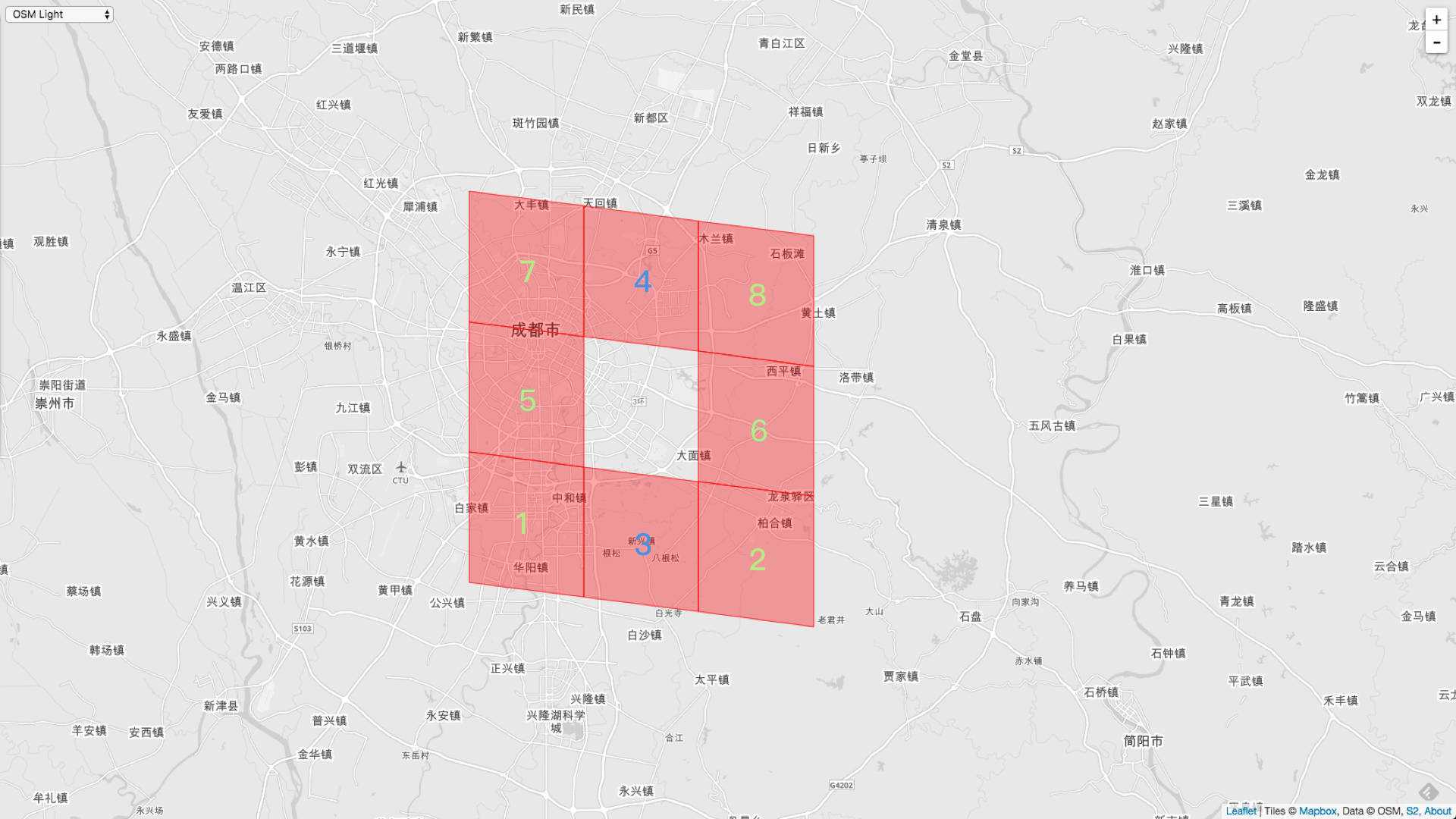``````
AllNeighbors := cellID.Parent(10).AllNeighbors(10)

3958601400295882752,
3958605798342393856,
3958603599319138304,
3958612395412160512,
3958599201272627200,
3958607997365649408,
3958623390528438272,
3958614594435416064

``````

``````
AllNeighbors := cellID.Parent(10).AllNeighbors(11)

3958600575662161920,
3958606622976114688,
3958603324441231360,
3958611570778439680,
3958600025906348032,
3958607172731928576,
3958603874197045248,
3958613220045881344,
3958599476150534144,
3958608821999370240,
3958623115650531328,
3958613769801695232

``````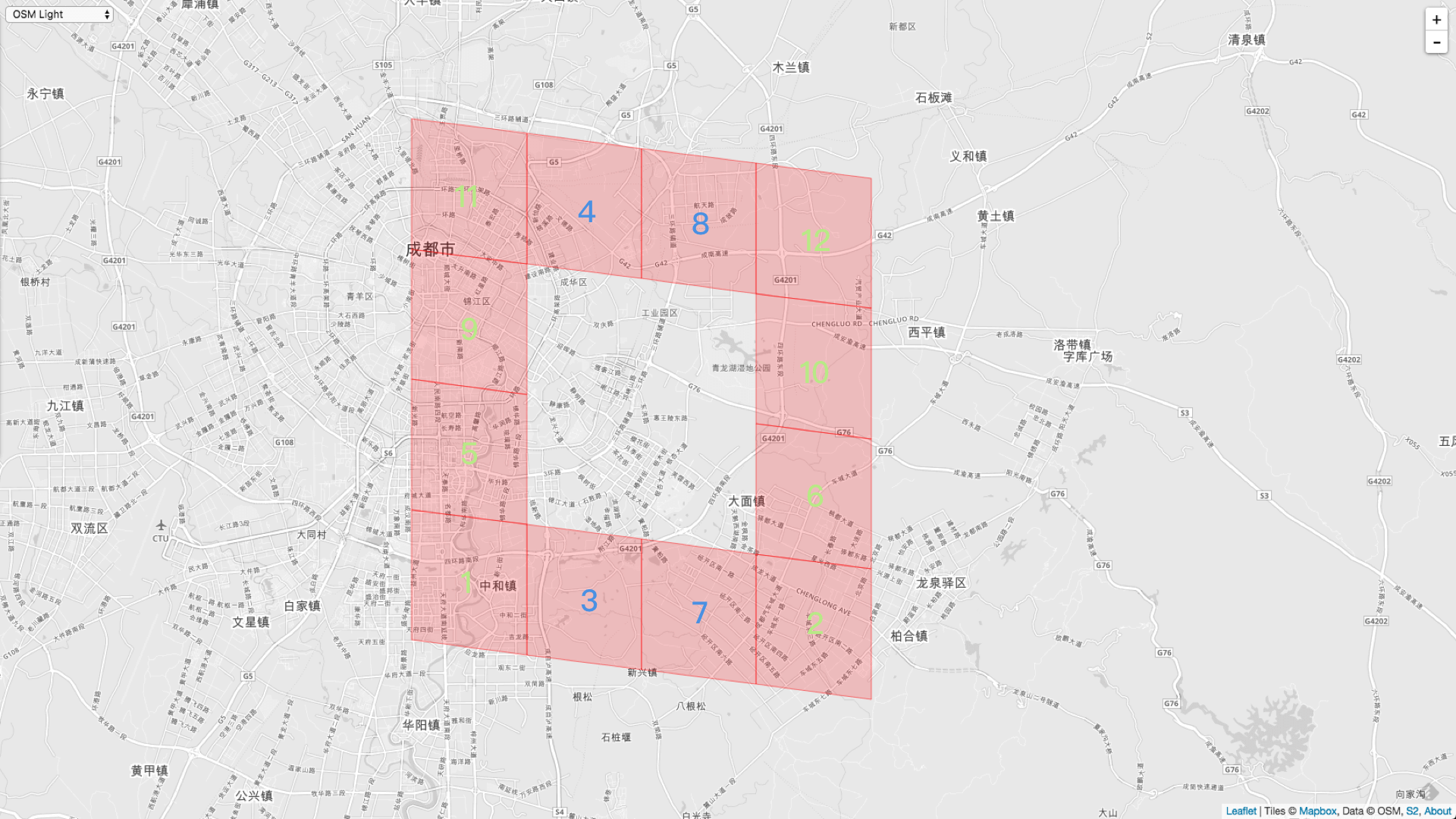1，2，5，6，9，10，11，12 都是左右邻居，3，4，7，8 是上下邻居。我们可以看到左右邻居是从下往上生成的。上下邻居是从左往右生成的。``````
AllNeighbors := cellID.Parent(10).AllNeighbors(9)

3958589305667977216,
3958580509574955008,
3958580509574955008,
3958615693947043840,
3958598101760999424,
3958606897854021632,
3958624490040066048,
3958615693947043840

``````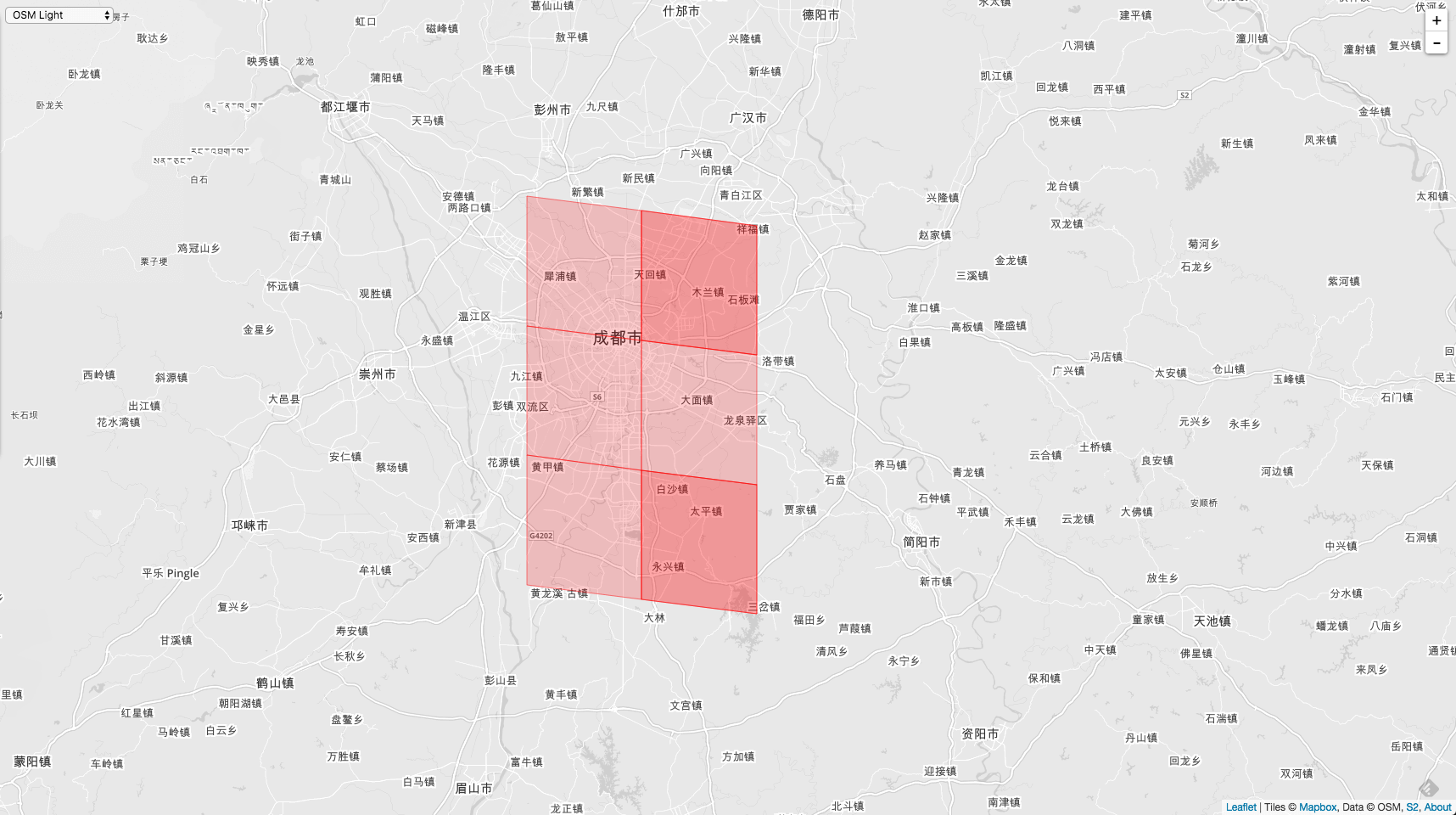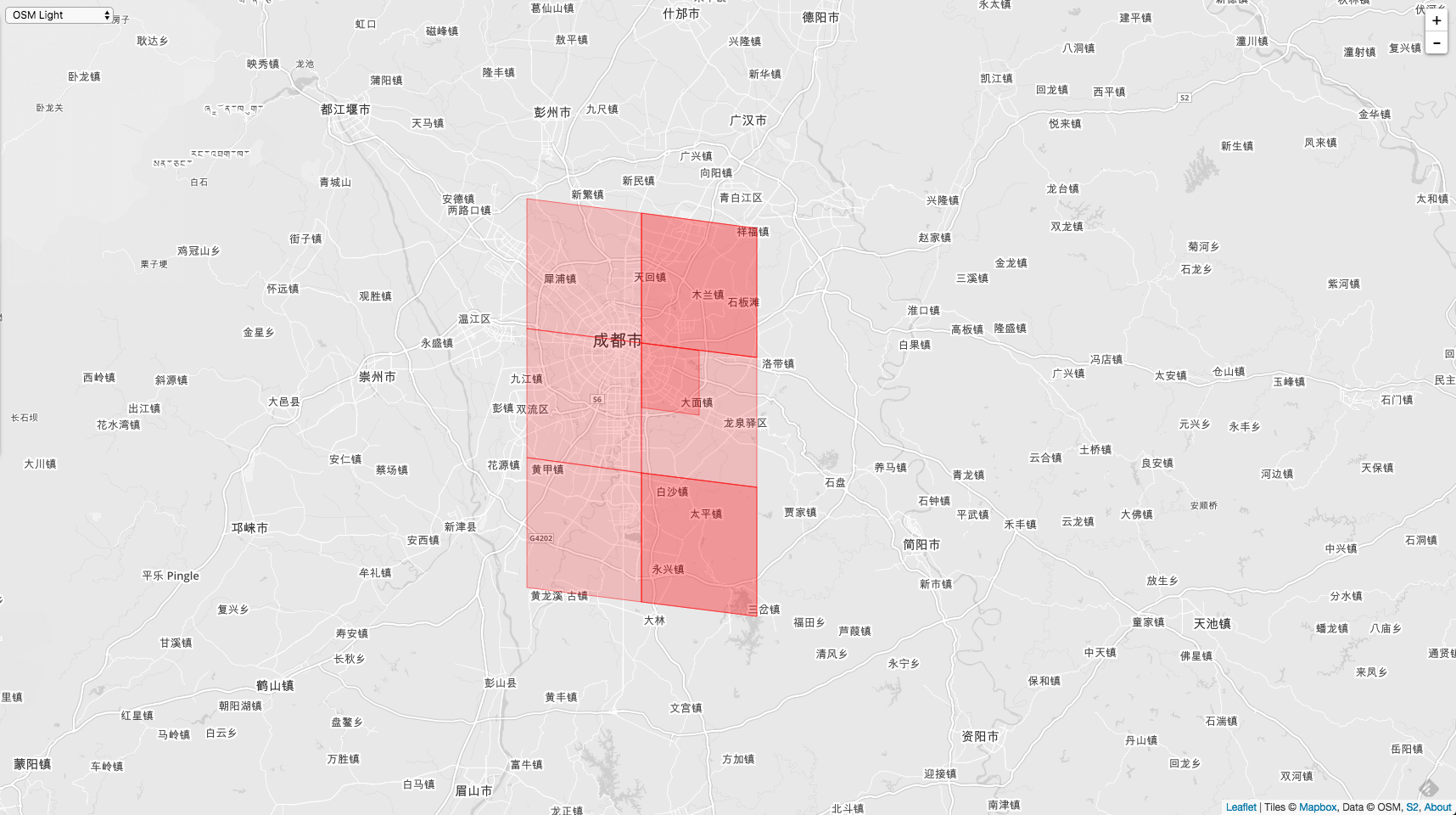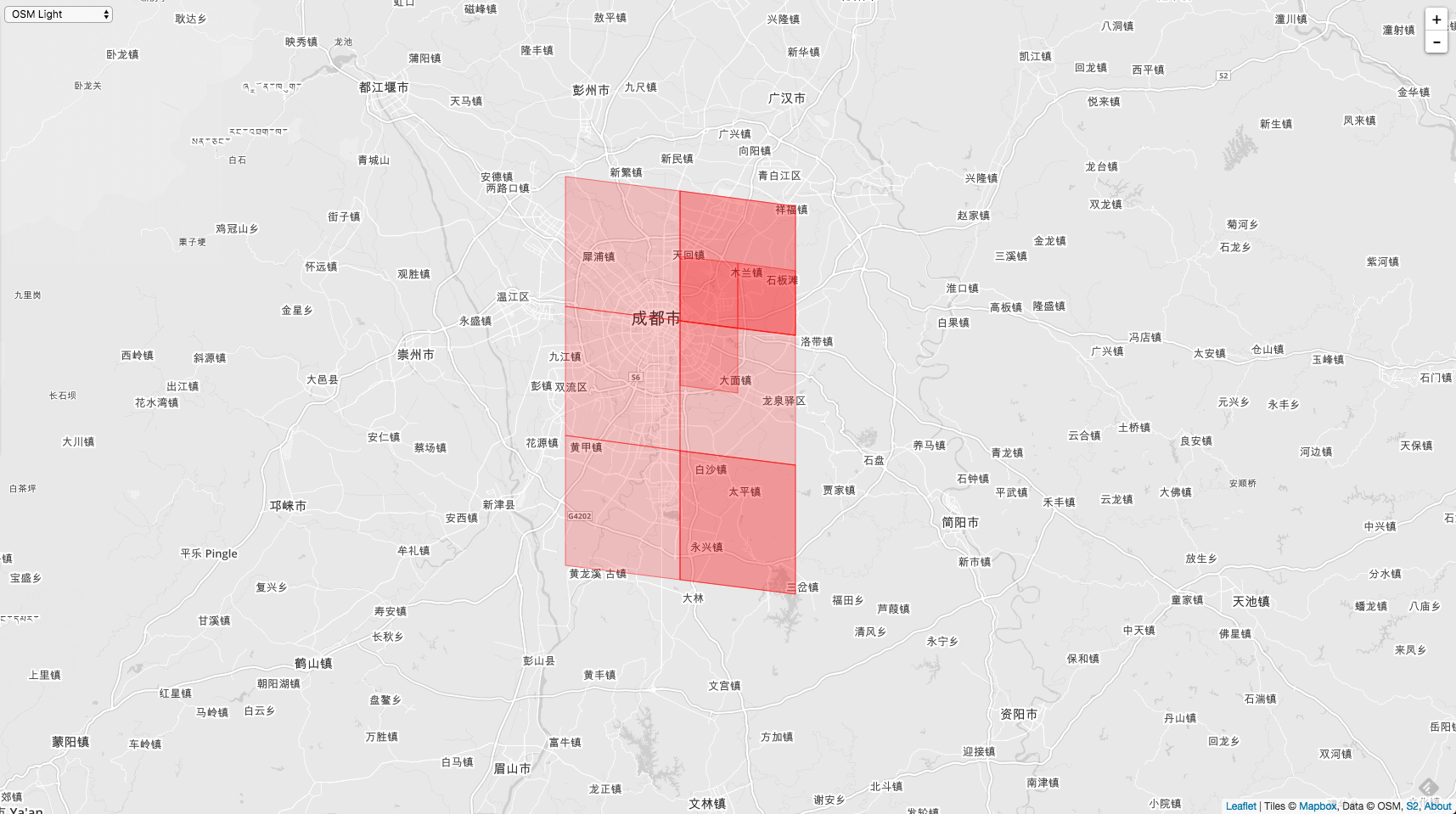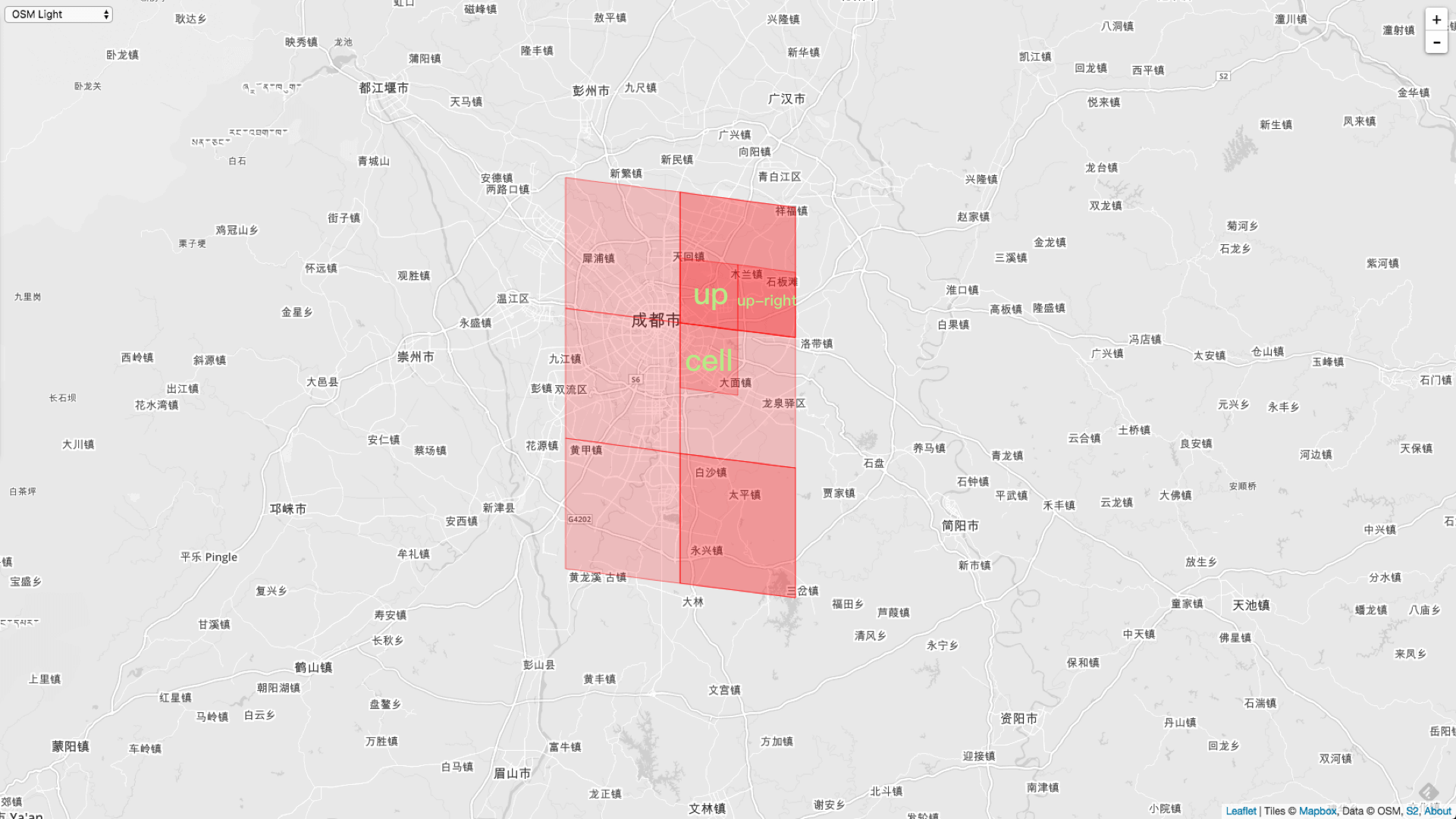``````
AllNeighbors := cellID.Parent(10).AllNeighbors(5)

3953034572924452864,
3946279173483397120,
3946279173483397120,
3957538172551823360,
3955286372738138112,
3957538172551823360,
3962041772179193856,
3959789972365508608

``````GitHub Repo：Halfrost-Field

Follow: halfrost · GitHubPrevious Post

Next Post